﻿ leetcode刷穿二叉树(六)(终章)
﻿• 价格透明
• 信息保密
• 进度掌控
• 售后无忧# leetcode刷穿二叉树(六)(终章)

### 终于在第六天拿下了二叉树# 这里有leetcode题集分类整理！！！

#### 今日目标：

• leetcode 669. 修剪二叉搜索树
• leetcode 108. 将有序数组转换为二叉搜索树
• leetcode 538. 把二叉搜索树转换为累加树
1. 修剪二叉搜索树
题目难度：中等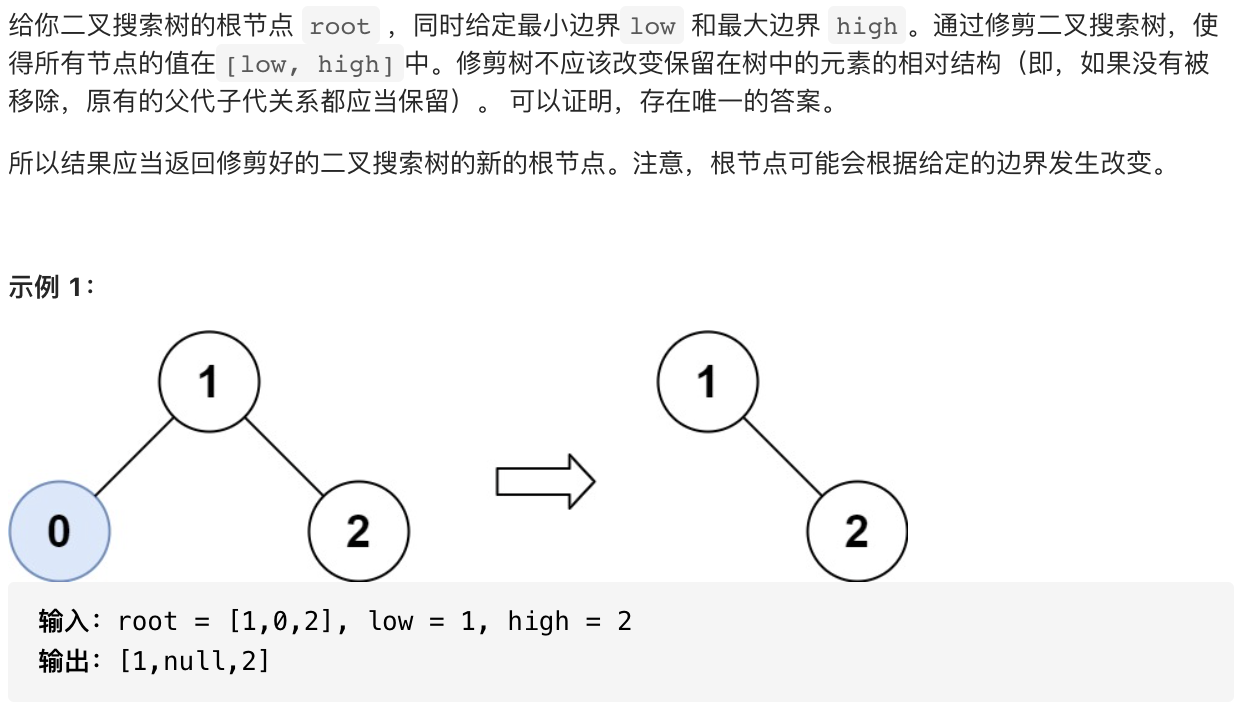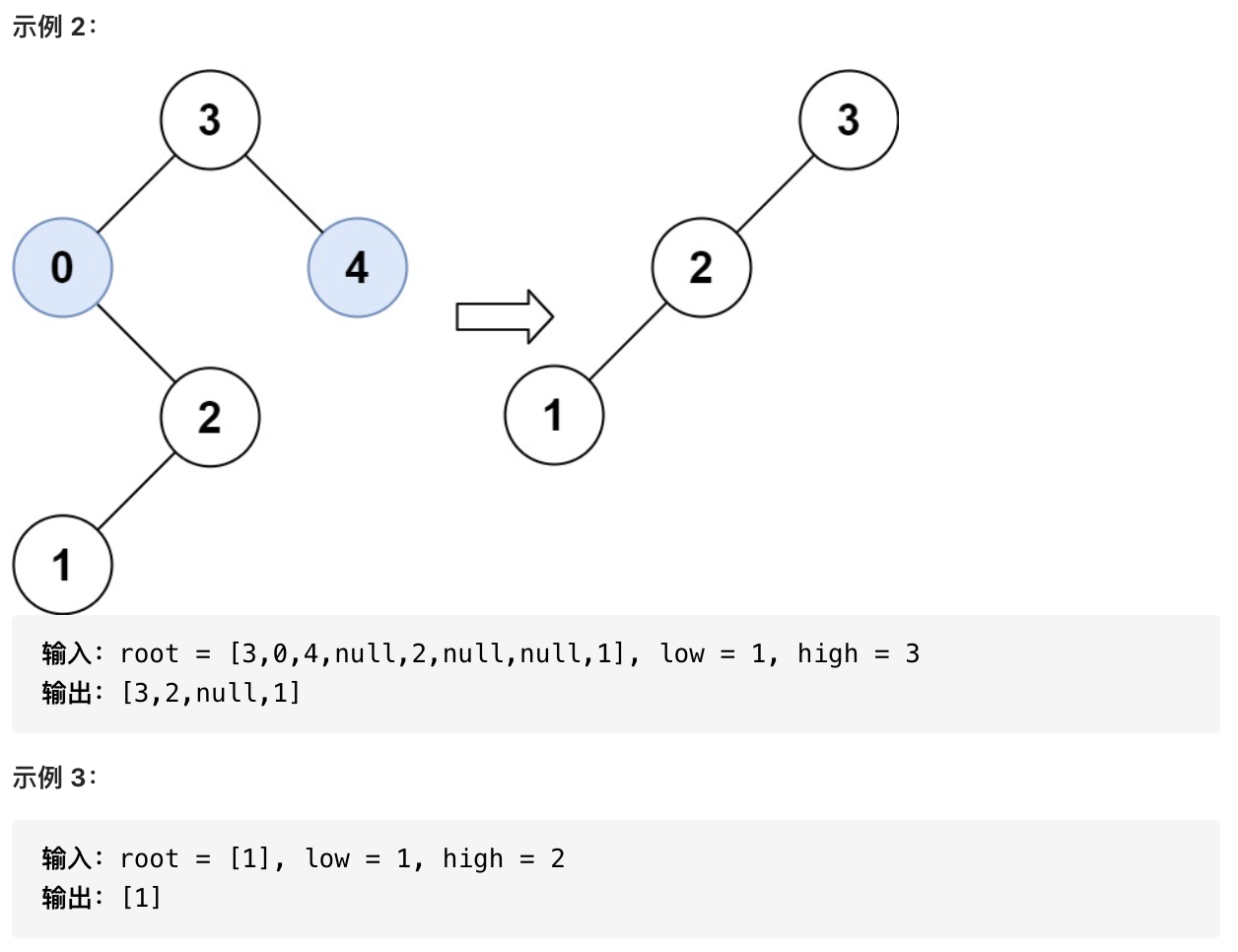解题思路：

`root.val` < `low``root.val` > `high`

• 每次递归返回符合条件的节点
• 将父节点与符合条件的节点进行重新组合返回
``````class Solution {
public TreeNode trimBST(TreeNode root, int low, int high) {
if (root == null) return null;
if (root.val < low) return trimBST(root.right, low, high);
if (root.val > high) return trimBST(root.left, low, high);
root.left = trimBST(root.left, low, high);
root.right = trimBST(root.right, low, high);
return root;
}
}
``````
1. 将有序数组转换为二叉搜索树
题目难度：简单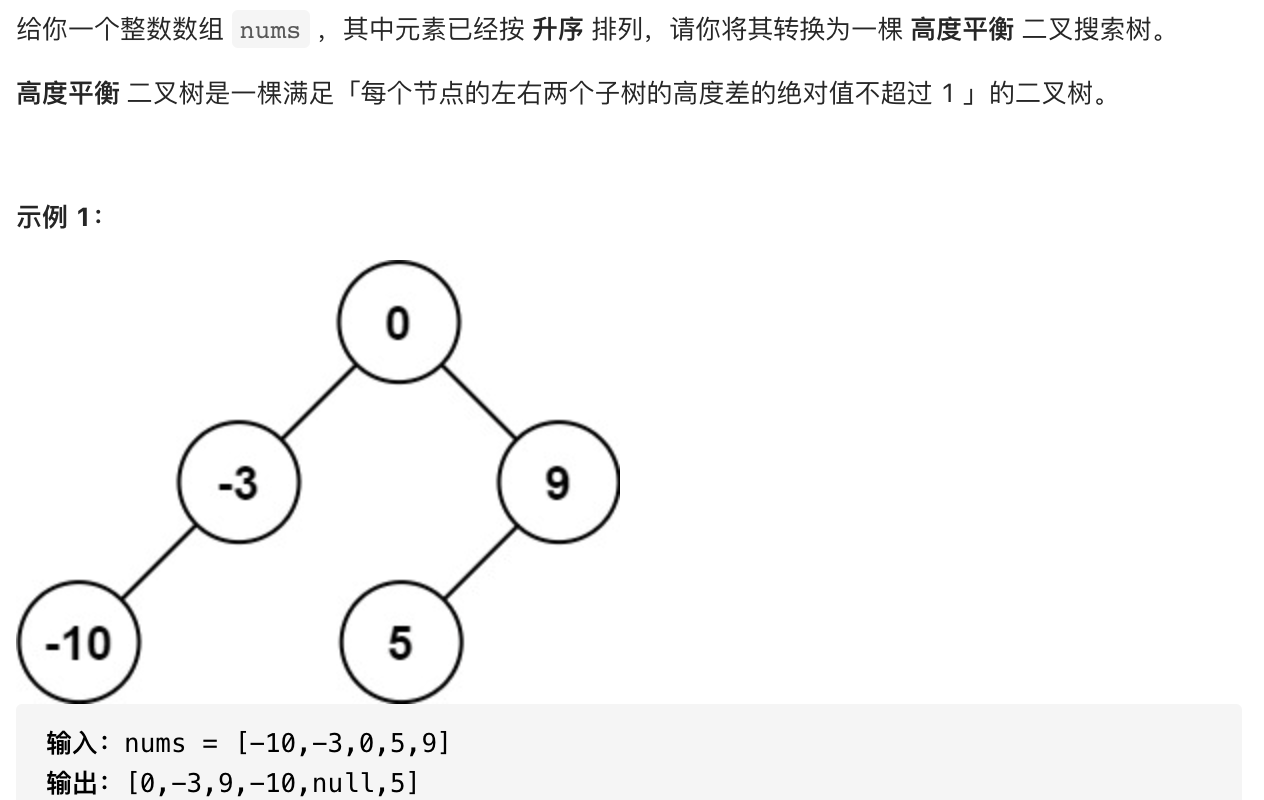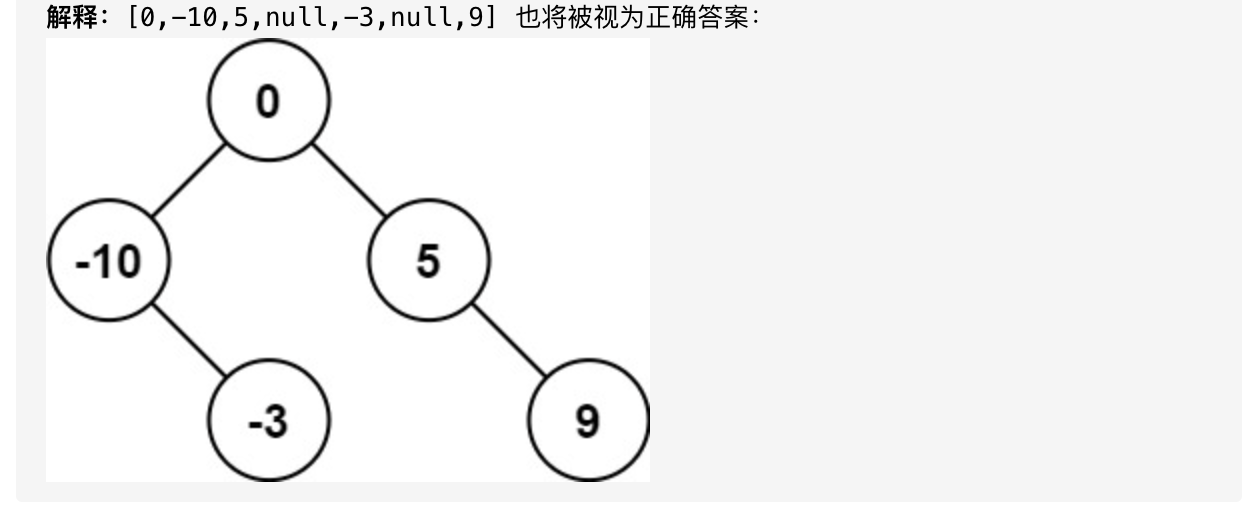解题思路：

• 用于确定孩子的数组左右边界相同，对应代码中的 `left` == `right`

• 计算当前数组范围的`rootIndex`
• 分别计算孩子节点位于数组的范围
• 左右孩子同时递归对应的`construct(nums, left, right)`
``````class Solution {
public TreeNode sortedArrayToBST(int[] nums) {
int n = nums.length;
return construct(nums, 0, n);
}

public TreeNode construct(int[] nums, int left, int right) {
// 数组为空返回
if (left == right) return null;
int rootIndex = left + right >> 1;
TreeNode node = new TreeNode(nums[rootIndex]);
// 递归创建节点
node.left = construct(nums, left, rootIndex);
node.right = construct(nums, rootIndex + 1, right);
// 返回节点
return node;
}
}
``````
1. 把二叉搜索树转换为累加树
题目难度：中等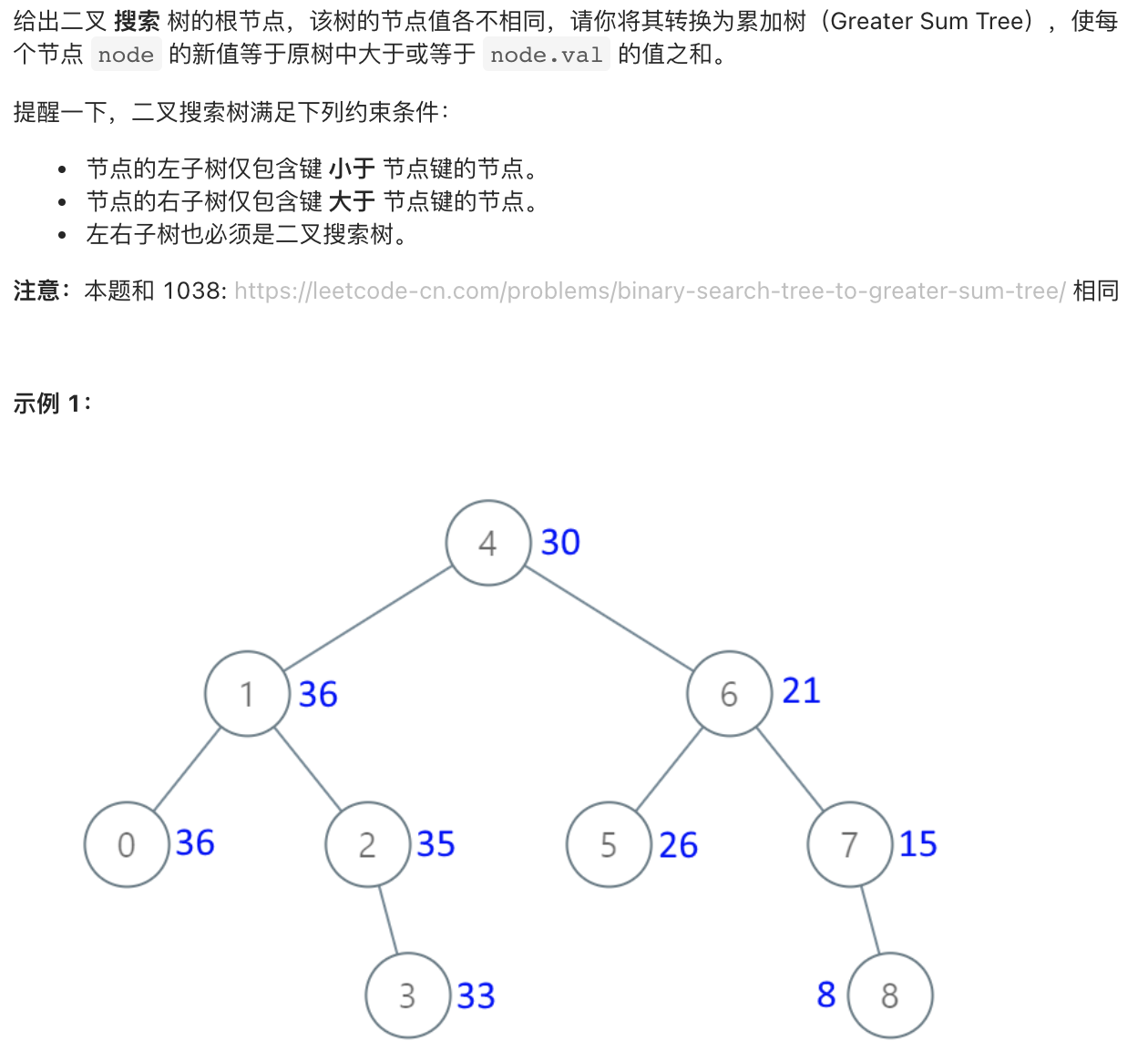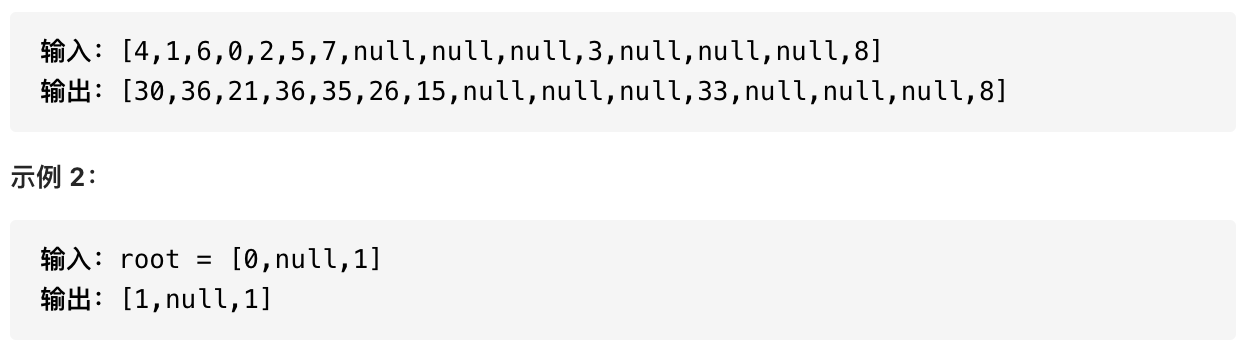解题思路一（朴素）：

• 中序遍历，将二叉树的数据遍历存储到数组（因为是BST，所以数组为升序数组）
• 将数组的值进行倒序叠加，使得数组编程降序的累加和。
• 中序遍历BST，对树上的值进行更新。

``````class Solution {
private int[] nums = new int;
private int count = 0;
public TreeNode convertBST(TreeNode root) {
inOrderTraversal(root);
calcNums();
count = 0;
update(root);
return root;
}

public void inOrderTraversal(TreeNode root) {
if (root == null) return;
inOrderTraversal(root.left);
nums[count ++] = root.val;
inOrderTraversal(root.right);
}

public void calcNums() {
for (int i = count - 1; i >= 0; i --) {
nums[i] = nums[i] + nums[i + 1];
}
}

public void update(TreeNode root) {
if (root == null) return;
update(root.left);
root.val = nums[count ++];
update(root.right);
}
}
``````

``````class Solution {
private int sum = 0;
public TreeNode convertBST(TreeNode root) {
if (root != null) {
convertBST(root.right);
sum += root.val;
root.val = sum;
convertBST(root.left);
}
return root;
}
}
``````### 低价透明### 金牌服务### 信息保密